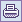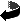# Gödel Theorems

## Paolo Caressa (2002)

The most famous, discussed and misunderstood theorems in Mathematics are Gödel incompleteness theorems (but do they really belong to Mathematics rather than Logic?): completeness theorem (which fully belongs to Logic, being its mathematical version the maximal ideal theorem for Boolean algebras) is in general neglected although it's a cornerstone in logic (the main reason Logicians use almost exclusively 1st order predicative calculus) as well as the third great contribution given by Gödel to Mathematics and Logic, namely the proof of the consistency of the axiom of choice and of the continuum hypothesis from the remaining axioms of set theory, while all the world talks about incompleteness theorem.

Gödel is not a machine: my personal opinions on the (ab)use of Gödel theorems in "proving" the methaphysical equation minds=machines

## Mathematical Logic: books

There are a lot of handbooks and introductions to logic and recursion theory; I have choosen from the ones I have read and I found useful and pleasant to read:

Nagel-Newman: Gödel's Proof, New York U.P. 1958
For non mathematician still a valuable source of informations.
Crossley et al: What is Mathematical Logic, Oxford, 1971
Another book for non mathematician, which contains discussions on Gödel results in logic and set theory.
Cohen: Set theory and the continuum hypothesis, Benjamin, 1966
A small great book on set theory: it's essentially devoted to the detailed exposition of Cohen's proof of independence, but indeed it contains an excellent introduction to Logic, Set Theory and Gödel theorems.
Church: Mathematical Logic Princeton, 1951 [reprinted in paperback]
the XX century book on the subject; however it's an half-book, since its 2nd planned volume still lies in the limbo of possible worlds (this is a proof that Leibniz was wrong...); its introduction is in itself a learned essay and each part of the book is carefully annoted and completed with interesting exercises; unluckily set theory, higher order logics, recursion theory and Gödel theorems were to be included in the 2nd volume...
Kleene: Introduction to Metamathematics Van Nostrand, 1952;
A classic chiefwork, noteworthy for its part on recursion theory; the exposition of Gödel results is clear and deep.
Gödel On undecidable propositions of formal mathematical systems, Princeton, 1934, [reprinted in Davies The Undecidable]
This is not the original paper on incompleteness theorem (which dates back to 1931) but it is a pleasant, clear and definitive exposition; here Gödel works in the second order logic, which makes thing more intuitive and appealing for a mathematician.
Gödel: The consistency of the axiom of choice and of the generalized continuum hypothesis with the axioms of set theory Princeton, 1940
This is a small book devoted to the proof of Gödel consistency theorem: it is the occasion for Gödel to reformulate Bernays set theory (which was in turn inspired by von Neumann's) according to his viewpoint; this version of set theory remains still the most used (as far as classes are concerned); difficult to read if you are not used to mathematics.
Manin: A Course on Mathematical Logic Springer, 1977
An elegant treatise addressed to mathematicians; the best medicine for mathematicians who do not like logic or believe that it's a philosophical nonsense (alas, there are...).

Maybe some reader is surprised from a missing item in the previous books list, namely that of the celebrated Hofstadter's book Gödel, Escher, Bach: reasons for such an exclusion are explained in my note Gödel is not a machine, which arise as a critique of Hofstadter's book and in which I express my feelings on the theme mind&machines.

## Completness Theorem

This is the theorem which guarantees that 1st order predicative calculus (thus propositional logic+quantification theory) is complete.

## Incompleteness Theorem

These are a couple of assertions about 1st order arithmetics (but indeed they do apply to each higher order mathematical theory and to other 1st order theories as well), saying that from axioms and inference rules one can't prove all arithmetical truths.

Some false propositions around Gödel theorem:

• Gödel proved that each mathematical theory is incomplete: this is false, for example Pressburger arithmetics, or Tarski's real numbers theory are complete.
• Gödel proved that there are mathematical proposition which are true but can't be proved: this is false because one can add axioms or generalize the theory in order to prove the theorem; a lot of progresses in mathematics were done exactly in this way (think to the early theory of rings, invented by Dedekind, Legendre et al. to prove Fermat last theorem).
• Gödel proved that one can't prove the consistency of Arithmetics: this is false, because Gerhard Gentzen for example gave a proof of consistency; the point is that this proof isn't (and indeed can't be, just for Gödel theorems) arithmetical: it uses transfinite induction.

Often one reads comments like the following one: Arithmetics (and every enough powerful mathematical theory) can't be perfect because it's incomplete.

I think that, for a mathematical theory to be incomplete is a merit, and not a fault: first of all a complete theory requires no great investigators, but simple craftsmen of symbolic manipulation to discover its truths; take for instance propositional calculus; this is the theory set up by logical connectives ("and", "or", "not", "implies", and so on: it sufficies the "not x nor y" connective for example to build the all theory) and its theorems may all be derived from a few axioms and a couple of inference rules (substitution and modus ponens); one can write a good computer program to perform this task; moreover propositional calculus is decidable, thus there exists a symbolic device to check whether a formula is true or not; for example using truth tables. So theorems of propositional calculus have nothing exciting, since their trueness may be verified by a computation.

So, for a theory: complete implies boring and decidable implies trivial.

If you do not agree think to this example: suppose you want to verify that 1911 is divisible by 7 (thus that there exists an integer number x such that x×7=1911); this is a matter of a computation; nobody will call it a theorem; if a theory is decidable then all of its theorems can be proved by computations like that.

Of course if you wonder whether 4294967297 is divisible by 641 then with a huge computation again you'll find that this is true (after some time...); however this fact is known since 1732, because the great mathematician Leonard Euler proved it: I mean instead of myriads of pages of computations, he provided a reasoning which proved that 641 actually divides 4294967297.

This is the difference between mechanical computations, and human mathematics: the less a theory is decidable, the more it's deep and challenging; the more the theory is complete, the less it will give us interesting informations.

Indeed when a theory is complete, I think its theorems are to be seen as analytical judgements (to use a kantian term), because the concept of truth is embodied in the concept of provability; on the other hand, incomplete theories have theorems which are in some sense synthetical judgements, since they are not implicit in axioms.

I think that the two good properties for a mathematical theory are not completeness and decidability, but semantic completeness (Henkin completeness) and categoricity; semantic completeness means that a given formula F of the theory is: 1) true in every model of the theory, or 2) false in every model of the theory. The second property (which implies the first), thus categoricity, means that all models are isomorphic (a model is a "possible world" in which formulas of the theory are natural laws; models are isomorphic if their individuals, functions, predicates, &c. can be put in one-to-one correspondence). Second order predicative calculus is complete in the sense of Henkin, for example [cfr. Church's book quoted in the suggested readings].

For example Arithmetics, the theory whose axioms are Peano's ones, framed in the 2nd order logic, is categorical and hence semantically complete: this means that, up to isomorphisms, there is only one model for natural numbers; this means that Peano axioms are the correct ones, which catch all essential properties of numbers.

First order Arithmetic, instead, is neither categorical, nor complete; the fact that it's not categorical should suggest that making number theory at the 1st order is not a good idea, since, numbers aside, one is talking about of a lot of exotic things (elements of non standard models).

And indeed mathematical theories are never at 1st order, but at order arbitrarily high: for example to define real numbers you need 4th order; think also about the following: consider the familiar (?!?) induction principle:

For each proposition P: if P(0) and (for each n: P(n) implies P(n+1)) then P(n) holds for every n.

This is a second order statement: we are saying "for all proposition P depending on a natural number", which is the same as saying "for every subset of the set of natural numbers" (indeed if X is a set of natural numbers that it determines a proposition "n belongs to X"; if P is a proposition it determines a set "the set of all n such that P(n) holds").

Now consider the 1st order version:

if P(0) and (for each n: P(n) implies P(n+1)) then P(n) holds for every n.

This is an axiom scheme, thus we obtain an axiom from it by substituting the occurrence of P with any valid formula of 1st order arithmetic; but these formulas are string of symbols (usually one uses a countable set of symbols, and the set of strings on a countable alphabet is countable), so they form a countable set: whence the second order version is much more powerful than the first order version, since for each subset of the natural numbers there's an induction statement, and those subsets are more than countable.

Indeed in every elementary algebra book it's proven that induction principle is equivalent to the fact that each subset of natural numbers has a first element: so the correct version of arithmetics is at least of second order; the same is true for all mathematical theories.

To conclude: I think that Gödel theorem tells us that arithmetics is a deep, interesting and challenging arena for human spirit. That's the great philosophical meaning of the result, according to me.

## Consistency Theorem

In 1938 Gödel gave his contribution to the question, the first of Hilbert's famous list, of the continuum; this was raised by Georg Cantor in 1877 [cf. Math.Annalen vol.21 (1883) pp.545-586]: Cantor discovered one of the most asthonishing things in XIX century thought, thus that there are different "levels" or "orders" of infinity; the first one corresponds to the "size" of the set of natural numbers: Cantor conjectured that the second "order of infinity" corresponds to the size of real numbers: this is the so called continuum hypothesis.

Another interesting question about set theory was the status of the axiom of choice: this was a proposition widely (and somewhat unawarely) used in XIX century analysis, and stated explicitely by Zermelo for the first time; many people thought about it as a complicated statement, too much cumbersome to be accepted as an axiom.

Now Gödel proved that both continuum hypothesis and axiom of choice are coherent with the other axioms of set theory: thus, if set theory is consistent, adding continuum hypothesis or axiom of choice (or both) we obtain another consistent theory. Later, in 1963, Paul Cohen proved that these axioms are also independent from set theory, thus even adding their negations to the theory (supposing the latter to be consistent) we get again consistent theories.

Gödel theorem is proved in his booklet; an introduction is given in Crossley's book and a complete proof in Cohen's book and Manin's book.Stampa questa paginaContatti: paolo(a)caressa.itHome: http://www.caressa.itChiudi